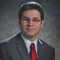# How to Calculate Depreciation

Calculating depreciation allows you to spread the cost of an asset over several years. Here's how to calculate depreciation using some common methods.

by Lance Cothern
updated May 31, 2022 ·  3min read

Depreciation is the process of spreading out the cost of an asset over its useful life. Companies generally choose to depreciate assets to deduct their cost over time, rather than all at once.

There are several methods for calculating depreciation, and in some circumstances, the Internal Revenue Service (IRS) or other outside groups may require businesses to use a specific one. Calculating depreciation can be straightforward or more complicated, depending on the method employed.## What You Must Know to Calculate Depreciation

Four main factors affect the depreciation of an asset:

• Cost of the asset
• Asset's useful life
• Salvage value
• Depreciation method

### Cost of the Asset

The cost of an asset, sometimes called the cost basis, is usually the price you paid for the item, including any sales taxes paid or discounts received. You can also include other costs associated with the purchase, such as shipping and installation charges.

### Asset's Useful Life

An asset's useful life is the length of time the item can function before it is no longer useable. In theory, the asset's useful life may be unique. However, accounting standards or IRS rules generally require you to set an asset's useful life at a standard length based on its classification. Here are some examples using classes from the IRS's general depreciation system.

• Five-year assets
• Cars
• Office machinery
• Appliances
• Seven-year assets
• Office furniture

### Salvage Value

An asset's salvage value is its estimated value when it reaches the end of its useful life.

### Depreciation Method

The depreciation method you use depends on the specific purpose for depreciating the item. Depreciation methods include:

## Calculating Depreciation the Easy Way

The easiest way to calculate depreciation is by using accounting software or a depreciation calculator. All you need to do is input information about the asset. Then, the software displays a depreciation schedule you can use to depreciate the asset.

## Manually Calculating Depreciation

You can also calculate depreciation manually. The straight-line depreciation method is the easiest to understand and implement. Start by subtracting the asset's salvage value from its cost. Then, divide the remaining amount by the asset's useful life. This gives you the amount of depreciation to recognize for each period.

Your business purchases an \$8,000 desk. You expect the desk's salvage value to be \$1,000 at the end of its useful life. This means the asset will depreciate by \$7,000. Since the desk is office furniture, the IRS defines its useful life as seven years. The seven-year life results in annual depreciation of \$1,000 using the straight-line depreciation method.

## Other Methods of Calculating Depreciation

Calculating other depreciation methods by hand is more involved. The double-declining balance method, for example, lets you write off more of an asset's value earlier in its useful life. Here, the percent depreciated each year is twice the rate of the straight-line method, applied to the asset's current value. For instance, you would depreciate a five-year asset by 40% of the asset's current cost basis each year—rather than 20% of the initial cost basis for straight-line depreciation—until its cost basis is equal to its salvage value.

Your company purchases a \$25,000 car that will have a \$5,000 salvage value at the end of its five-year useful life. You'd depreciate the asset by 40% of \$25,000, or \$10,000, in the first year. In year two, the asset's remaining cost basis is \$15,000, so the second-year depreciation would be \$6,000, or 40% of \$15,000. In year three, you would depreciate the asset by \$3,600, or 40% of \$9,000.

At the beginning of year four, the asset has a remaining cost basis of \$5,400. Depreciating the asset by another 40% would bring the asset's value below its \$5,000 salvage value. Instead, only depreciate the remaining amount to get to the asset's salvage value. This results in a depreciation expense of \$400. You would not depreciate the item in year five since its depreciated value already equals its cost basis.

Choosing the right depreciation method depends on your situation. Regulations may require you to use a particular type of depreciation. If they don't, you can choose the depreciation method that works best for your circumstances.